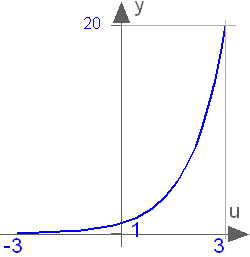# 'exp()'

exp()# Information

This information is part of the Modelica Standard Library maintained by the Modelica Association.

Exponential, base e.

`exp(u)`

#### Description

Returns the base e exponential of u, with -∞ < u < ∞ Argument u needs to be an Integer or Real expression.

The exponential function can also be accessed as Modelica.Math.exp.#### Examples

```exp(1)
= 2.71828182845905```